# KSEEB Solutions for Class 8 Maths Chapter 3 Understanding Quadrilaterals Ex 3.3

You can Download KSEEB Solutions for Class 8 Maths Chapter 3 Understanding Quadrilaterals Ex 3.3 Questions and Answers helps you to revise the complete syllabus.

## KSEEB Solutions for Class 8 Maths Chapter 3 Understanding Quadrilaterals Ex 3.3

Question 1.
Given a parallelogram ABCD. Complete each statement along with the definition or property used.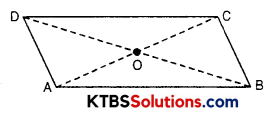(ii) ∠DCB = ___________
(iii) OC = ___________
(iv) m∠DAB + m∠CDA = ___________
Solution:
(i) AD = BC (opposite sides of a parallelogram are equal)
(ii) ∠DCB = ∠DAB (opposite angles of a || gm are equal)
(iii) OC = OA (Diagonals of a ygin bisect each other)
(iv) m∠DAB + m∠CDA = 180° (Sum of cointerior angles of a y gm between AB y CD)Question 2.
Consider the following parallelograms. Find the values of the unknown x, y, z.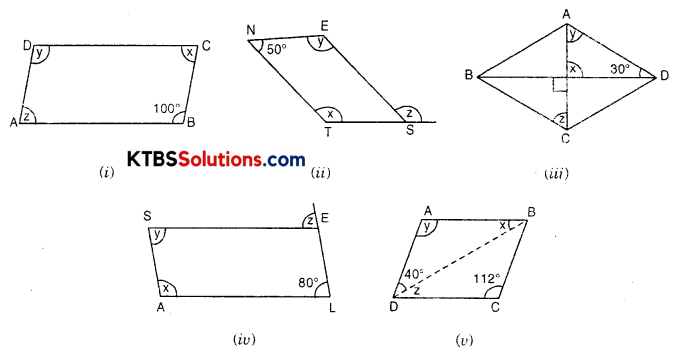Solution:
(i) ∠D = ∠B (opposite angles of a || gm are equal)
∴ y = 100° [∠B = 100°]
In || gm ABCD
∠y + ∠z = 180° (Sum of cointerior angles between AB || CD)
⇒ 100° + ∠z = 180°
⇒ ∠z = 180° – 100°
⇒ ∠z = 80°
∠z = ∠x (opposite angles of || gm are equal)
∴ ∠x = 80°
Hence ∠A = ∠z = 80°, ∠D = ∠y = 100°, ∠C = ∠x = 80°

(ii) NEST is a parallelogram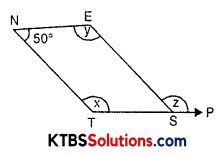∴ ∠N = ∠TSE (opposite angles of a || gm)
⇒ 50° = ∠TSE
∠TSE + ∠z = 180° (Linear pair)
⇒ 50° + ∠z = 180°
⇒ ∠z = 180° – 50°
⇒ ∠z = 130°
Now, in ygm NEST
∠N + ∠E + ∠S + ∠T = 360°
⇒ 50° + y + 50° + x = 360°
⇒ x + y + 100° = 360°
⇒ x + y = 360° – 100°
⇒ x + y = 260°
But ∠x = ∠y (opposite angles of a || gm)
⇒ x + x = 260°
⇒ 2x = 260°
⇒ x = 130°
∠N = 50°, ∠E = y = 130°, ∠S = 50°, ∠T = x = 130°, z = 130°(iii) ∠AOD = ∠BOC (Vertically opposite angles)
∠AOD = 90°
In ∆AOD
⇒ y + x + 30° = 180°
⇒ y + 90° + 30° = 180°
⇒ y = 180° – 120° = 60°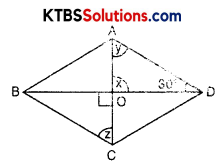⇒ 60° = ∠OCB
⇒ 60° = z

(iv) SALE is a || gm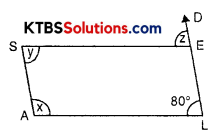∴ ∠S = ∠L (opposite angles of a || gm)
y = 80°
∠SED = ∠L (corresponding angles)
z = 80°
∠A + ∠L = 180° (coniterior angles between AS || LE)
⇒ x + 80° = 180°
⇒ x = 180° – 80° = 100°
∴ x = 100°, y = 80°, z = 80°
(v) ABCD is a ygm
∠A = ∠C (opposite angles of a || gm)
⇒ y = 112°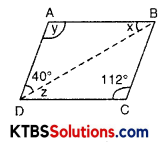In ∆ABD,
∠A + ∠ABD + ∠ADB = 180°
⇒ 112° + x + 40° = 180°
⇒ 152° + x = 180°
⇒ x = 180° – 152°
∴ x = 28°
∠ABD = ∠BDC (alternate angles)
⇒ x = z
⇒ 28° = z
∴ x = 28°, y = 112°, z = 28°

Question 3.
Can a quadrilateral ABCD be a parallelogram, if
(i) ∠D + ∠B = 180°?
(ii) AB = DC = 8 cm, AD = 4 cm and BC = 4.4 cm?
(iii) ∠A = 70° and ∠C = 65°?
Solution:
(i) It can be a parallelogram in the case of rectangle and square. Since the sum of opposite angles in both of these is 180° and these are parallelograms as well. But in another case when a quadrilateral is a parallelogram, it need not be, that sum of opposite angles is 180°.

(ii) No, it is not a parallelogram. In a parallelogram both pairs of opposite sides are equal. But here AB ≠ DC and AD ≠ BC.

(iii) ∠A ≠ ∠C
∴ It is not a parallelogram.
In parallelogram opposite angles are equal.Question 4.
Draw a rough figure of a quadrilateral that is not a parallelogram but has exactly two opposite angles of equal measure.
Solution: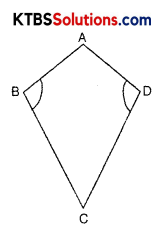The figure of the kite, in which ∠B = ∠D, but it is not a parallelogram.

Question 5.
The measures of two adjacent angles of a parallelogram are in the ratio 3 : 2. Find the measure of each of the angles of the parallelogram.
Solution: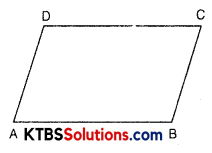Let the ratio be x.
∴ angle measure 3x and 2x
∴ Let ∠D = 3x and ∠A = 2x
But ∠A + ∠D = 180° (Sum of adjacent angles in a || gm)
⇒ 2x + 3x = 180°
⇒ 5x = 180°
∴ x = $$\frac{180^{\circ}}{5}$$ = 36°
∴ ∠A = 2 x 36° = 72°
∠D = 3 x 36° = 108°
But ∠D = ∠B and ∠A = ∠C
∴ 108° = ∠B and 72° = ∠C

Question 6.
Two adjacent angles of a parallelogram have equal measure. Find the measure of each of the angles of the parallelogram.
Solution: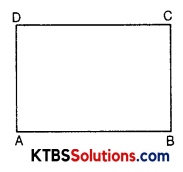ABCD is a parallelogram
∠A = ∠D = x (say) [∵ Adjacent angles are equal]
But ∠A + ∠D = 180° (Sum of adjacent angles in a || gm is 180°)
⇒ x + x = 180°
⇒ 2x = 180°
∴ x = 90°
∴ ∠A = 90° = ∠D
But ∠A = ∠C and ∠B = ∠D
∴ ∠A = ∠B = ∠C = ∠D = 90°Question 7.
The adjacent figure HOPE is a parallelogram. Find the angle measures x, y, and z. State the properties you use to find them.
Solution: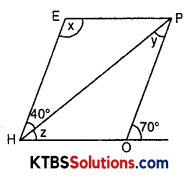HOPE is a parallelogram
70° + ∠HOP = 180° (linear pair)
⇒ ∠HOP = 180° – 70°
⇒ ∠HOP = 110°
∠E = ∠HOP (opposite angles of a || gm)
⇒ ∠E = 110°
∴ x = 110°
In ∆EPH,
∠E + ∠EHP + ∠EPH = 180°
⇒ x + 40° + ∠EPH = 180°
⇒ 110° + 40° + ∠EPH = 180°
⇒ ∠EPH = 180° – 150°
∴ ∠EPH = 30°
∠EPH = ∠PHO (Alternate angls)
⇒ 30° = ∠PHO
∴ z = 30°
In ∆OPH,
z + y + ∠HOP = 180°
⇒ 30° + y + 110° = 180°
⇒ y = 180° – 140°
∴ y = 40°
∴ x = 110°, y = 40°, z = 30°

Question 8.
The following figures GUNS and RUNS are parallelograms. Find x and y. (Lengths are in cm)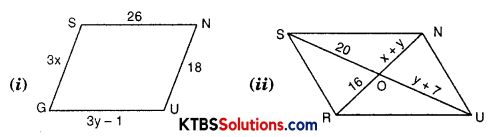Solution:
GUNS is a parallelogram
GU = SN (opposite sides of a || gm are equal)
⇒ 3y – 1 = 26
⇒ 3y = 26 + 1
⇒ 3y = 27
∴ y = 9
Now, GS = NU
⇒ 3x = 18
⇒ x = 6(ii) RUNS is a parallelogram.
SU and RN are diagonals.
Since, diagonals of a parallelogram bisect each other.
∴ OS = OU
⇒ 20 = y + 7
⇒ 20 – 7 = y
⇒ y = 13
Again OR = ON
⇒ 16 = x + y
⇒ 16 = x + 13
⇒ 16 – 13 = x
∴ 3 = x

Question 9.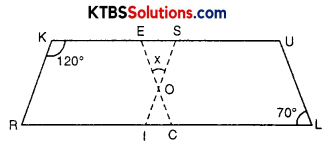In the above figure both RISK and CLUE are parallelograms. Find the value of x.
Solution:
RISK is a parallelogram
∠K = ∠RIS (opposite angles of a || gm)
120° = ∠RIS
∠KSI + ∠RIS = 180° (Sum of adjacent angles in a || gm)
⇒ ∠KSI + 120° = 180°
⇒ ∠KSI = 180° – 120°
∴ ∠KSI = 60°
Again, CLUE is a parallelogram
∠CEU = ∠L (opposite angles of a || gm)
⇒ ∠CEU = 70°
Now, in ∆OES
∠O + ∠E + ∠S = 180°
⇒ x + 70° + 60° = 180°
⇒ x + 130° = 180°
⇒ x = 180° – 130°
∴ x = 50°

Question 10.
Explain how this figure is a trapezium. Which of its two sides is parallel?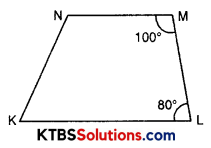Solution:
∠L + ∠M = 180° (Given)
But, these are adjacent angles and their sum is 180°.
Hence, MN || KL
So, KLMN is a trapezium.
In a trapezium, one pair of opposite sides of a quadrilateral are parallel.Question 11.
Find m∠C in Fig if $$\overline{A B}$$ y $$\overline{D C}$$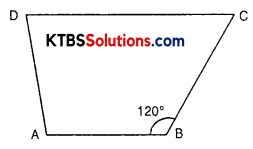Solution:
ABCD is a quadrilateral and AB || CD
∴ m∠C + m∠B = 180°(Sum of angles between two parallel lines)
⇒ m∠C + 120° = 180°
⇒ m∠C = 180° – 120°
∴ m∠C = 60°

Question 12.
Find the measure of ∠P and ∠S, if $$\overline{S P}$$ y $$\overline{R Q}$$ in Fig. (If you find m∠R, is there more than one method to find m∠P?)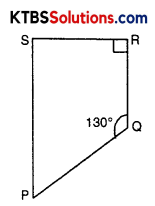Solution:
PQRS is a quadrilateral in which ∠Q = 130° and ∠R = 90° and SP || RQ
∠P + ∠Q = 180° (Sum of adjacent angles between two parallel lines i.e., SP || RQ)
⇒ ∠P + 130° = 180°
⇒ ∠P = 180° – 130°
∴ ∠P = 50°
Similarly, ∠S + ∠R = 180°
⇒ ∠S + 90° = 180°
⇒ ∠S = 180° – 90°
∴ ∠S = 90°